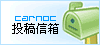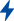## 西藏航空冬春航季新开十条航线

2017-10-27 21:04:30 来源：民航资源网 作者：通讯员冉梦雪 　[投稿排行榜]

民航资源网2017年10月27日消息：西藏航空将于10月29日正式执行冬春航季时刻，新航季对原有航线布局进行了一定的调整，开通了10条商务旅行热门航线，西安=拉萨=阿里、成都=洛阳、拉萨=玉树=西宁、兰州=济南、沈阳=郑州、拉萨=贵阳=南昌、兰州=丽江=南昌、成都=铜仁=福州、成都=铜仁=温州、昆明=林芝=拉萨等航线。航线覆盖总体原则是秉承在保持原有的航线的情况下，继续延伸出去，进一步提升航线、航点的通达性。

西藏航空作为国内首家高高原航空公司，立足西藏、辐射全国、内接国内、外联南亚是西藏航空的发展战略思路。根据航季的变化和市场需求的变化，新增南昌、洛阳、温州、福州航点。西藏航空A330宽体机将投入冬春旅游黄金线路成都=三亚以及公商务和旅游兼顾的成都=上海航线，这两条航线无论是在时刻的选取、机型的配置都采取了最佳的调配。西北地区新开独飞航线西安=拉萨=阿里航线，为进入后藏区域提供了更便捷的交通方式。新开的兰州=丽江=南昌和兰州=济南航线，也架起了从西北地区到西南地区和华东地区的空中桥梁。西南地区新开成都=铜仁=温州、成都=铜仁=福州、成都=洛阳、昆明=林芝=拉萨航线，恢复重庆=长沙、林芝=重庆=深圳、成都=杭州等航线。

需要了解更多相关信息请登陆西藏航空官方网站www.tibetairlines.com.cn或拨打西藏航空24小时客服热线4008-0891-88。

新增航班时刻如下（具体时刻，以系统查询为准）：

西安=拉萨=阿里

TV6017 西安06:00=09:35拉萨10:15=12:30阿里

TV6018 阿里13:10=14:55拉萨19:05=21:45西安

每日一班，由空客A319执飞

兰州=丽江=南昌

TV6021 兰州08:05=10:25丽江11:15=13:35南昌

TV6022 南昌14:25=17:05丽江17:55=20:05兰州

每日一班，由空客A320执飞

兰州=济南

TV6025 兰州17:55=20:05济南

TV6026 济南21:00=23:30兰州

每日一班，由空客A320执飞

拉萨=贵阳=南昌

TV6027 拉萨11:15=13:45贵阳14:35=16:10南昌

TV6028 南昌17:15=19:15贵阳20:10=23:25拉萨

每日一、三、五、七各一班班，由空客A319执飞

昆明=林芝=拉萨

TV6033 昆明07:40=10:20林芝11:10=12:15拉萨TV6034　拉萨09:35=10:30林芝11:10=13:25昆明

每日一班，由空客A319执飞

沈阳=郑州

TV9889 沈阳11:20=14:05郑州

TV9890 郑州17:25=19:50沈阳

每日一班，由空客A320执飞

成都=铜仁=温州

TV9897 成都15:40=17:00铜仁17:40=19:30温州

TV9898 温州20:20=22:40铜仁23:30=01:15+1成都

每周一、三、五、七各一班，由空客A319执飞

成都=铜仁=福州

TV9901 成都15:40=17:00铜仁17:40=19:30福州

TV9902 福州20:20=22:40铜仁23:30=01:15+1成都

每周二、四、六各一班，由空客A319执飞

成都=洛阳

TV9851 成都18:45=20:15洛阳

TV9852 洛阳22:40=00:50+1成都

每周三、五、七各一班，由空客A319执飞

拉萨=玉树=西宁

TV9931 拉萨13:20=14:45玉树15:35=16:50西宁

（供稿：西藏航空有限公司快讯更多